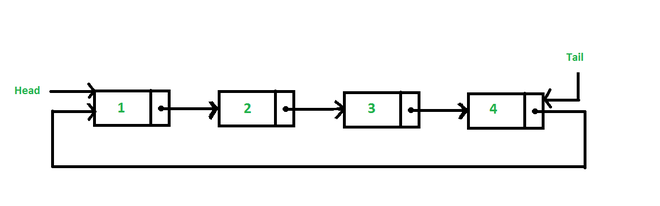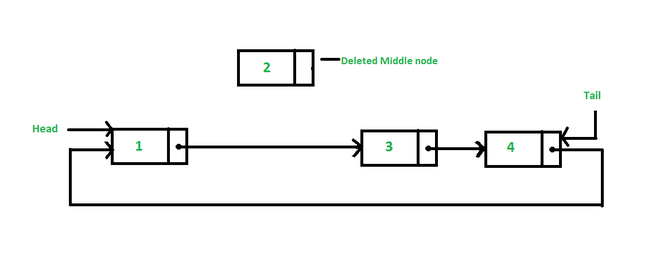Related Articles
Java Program to Delete a Node From the Middle of the Circular Linked List
• Difficulty Level : Medium
• Last Updated : 04 Dec, 2020

In this article, we are going to learn to delete the middle node from the circular Linked List in java. The approach we are going to follow for this program is, first we calculate the number of nodes in the list and then divide the number of nodes by 2 to get the middle node of the list.### Algorithm

Case 1: List is empty

• Then simply just return from the function.

Case 2: List has only one node

• It means head == tail then assigned head and tail equal to null.

Case 3: List has only two nodes

• It means the middle node of the list at 1 position. In this case, we have to modify the head and tail also. Head becomes tail and tail.next becomes also tail.

Case 4: List has more than two nodes

• It means the position of the middle node is greater than 1. In this case, we define the two-node temp and prev. Assign head to the temp.
• Then traverse the list using temp up to the middle node. In each iteration assign prev = temp and temp = temp.next
• Now temp is pointing to the middle node and prev pointing to the previous node of the middle node.
• Then simply set prev.next=temp.next and temp=null,

## Java

 `// Java Program to Delete a ``// Node From the Middle of the``// Circular Linked List`` ` `class` `CLinkedList {`` ` `    ``class` `Node {``        ``int` `data;``        ``Node next;``    ``}``    ``private` `static` `int` `size;`` ` `    ``// head points first node and``    ``// tail points to last node``    ``private` `Node head, tail;``    ``CLinkedList()``    ``{``        ``this``.head = ``null``;``        ``this``.tail = ``null``;``        ``size = ``0``;``    ``}``    ``public` `void` `addNode(``int` `d)``    ``{``        ``// create a empty node``        ``Node n = ``new` `Node();`` ` `        ``// list is empty``        ``if` `(``this``.head == ``null``) {``            ``n.data = d;``            ``this``.head = n;``            ``this``.tail = n;``            ``n.next = ``this``.head;``        ``}`` ` `        ``// list has one node or more than one nodes``        ``else` `{``            ``n.data = d;``            ``tail.next = n;``            ``tail = n;``            ``tail.next = head;``        ``}``        ``size++;``    ``}``    ``public` `void` `deleteNodeMiddle()``    ``{``        ``int` `loc;``        ``Node temp, prev;`` ` `        ``// calculating position of middle node``        ``if` `(size % ``2` `== ``0``) {``            ``loc = size / ``2``;``        ``}``        ``else` `{``            ``loc = (size / ``2``) + ``1``;``        ``}`` ` `        ``// list is empty``        ``if` `(head == ``null``) {``            ``return``;``        ``}`` ` `        ``// list contains only one node``        ``else` `if` `(head == tail) {``            ``head = ``null``;``            ``tail = ``null``;``        ``}`` ` `        ``// list contains only two nodes``        ``else` `if` `(loc == ``1``) {``            ``head = tail;``            ``tail.next = tail;``        ``}`` ` `        ``// list contains more than two nodes``        ``else` `{``            ``temp = head;``            ``prev = ``null``;``            ``int` `i = ``1``;``            ``while` `(i < loc) {``                ``prev = temp;``                ``temp = temp.next;``                ``i++;``            ``}``            ``prev.next = temp.next;``            ``temp = ``null``;``        ``}`` ` `        ``size--;``        ``if` `(size < ``0``) {``            ``size = ``0``;``        ``}``    ``}``    ``public` `void` `display()``    ``{``        ``// displaying list``        ``if` `(head == ``null``) {``            ``System.out.println(``"List is empty"``);``        ``}``        ``else` `{``            ``Node temp = head;``            ``do` `{``                ``System.out.print(temp.data + ``" "``);``                ``temp = temp.next;``            ``} ``while` `(temp != head);``            ``System.out.println();``        ``}``    ``}``}``class` `Test {``    ``public` `static` `void` `main(String args[])``    ``{``        ``CLinkedList c1 = ``new` `CLinkedList();`` ` `        ``// adding node in linkedlist``        ``c1.addNode(``10``);``        ``c1.addNode(``20``);``        ``c1.addNode(``30``);``        ``c1.addNode(``40``);`` ` `        ``// displaying nodes after adding``        ``System.out.print(``"List="``);``        ``c1.display();`` ` `        ``// delete node in middle``        ``c1.deleteNodeMiddle();`` ` `        ``// displaying linkedlist after deleting middle node``        ``System.out.print(``            ``"List after deleting middle node="``);``        ``c1.display();`` ` `        ``c1.deleteNodeMiddle();`` ` `        ``// displaying linkedlist after deleting middle node``        ``System.out.print(``            ``"List after deleting middle node="``);``        ``c1.display();`` ` `        ``c1.deleteNodeMiddle();`` ` `        ``// displaying linkedlist after deleting middle node``        ``System.out.print(``            ``"List after deleting middle node="``);``        ``c1.display();`` ` `        ``c1.deleteNodeMiddle();`` ` `        ``// displaying linkedlist after deleting middle node``        ``System.out.print(``            ``"List after deleting middle node="``);``        ``c1.display();``    ``}``}`
Output
```List=10 20 30 40
List after deleting middle node=10 30 40
List after deleting middle node=10 40
List after deleting middle node=40
List after deleting middle node=List is empty```

Attention reader! Don’t stop learning now. Get hold of all the important Java Foundation and Collections concepts with the Fundamentals of Java and Java Collections Course at a student-friendly price and become industry ready. To complete your preparation from learning a language to DS Algo and many more,  please refer Complete Interview Preparation Course.

My Personal Notes arrow_drop_up